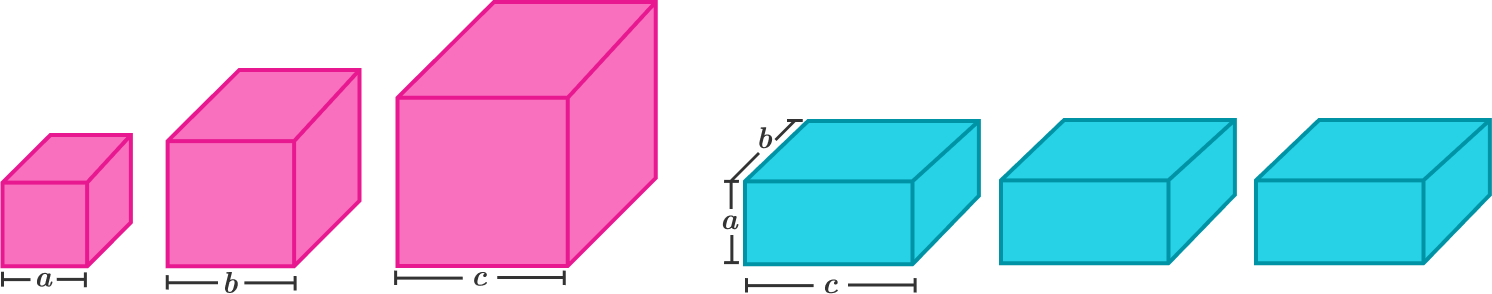# Present Mystery$a, b, c\ (a are coprime integers, and there are the following 2 sets of present boxes:

• $3$ pink cubic boxes of respective side lengths $a, b, c$
• $3$ identical blue cuboid boxes of dimensions $a\times b\times c$.

Which set has a larger total surface area?

×